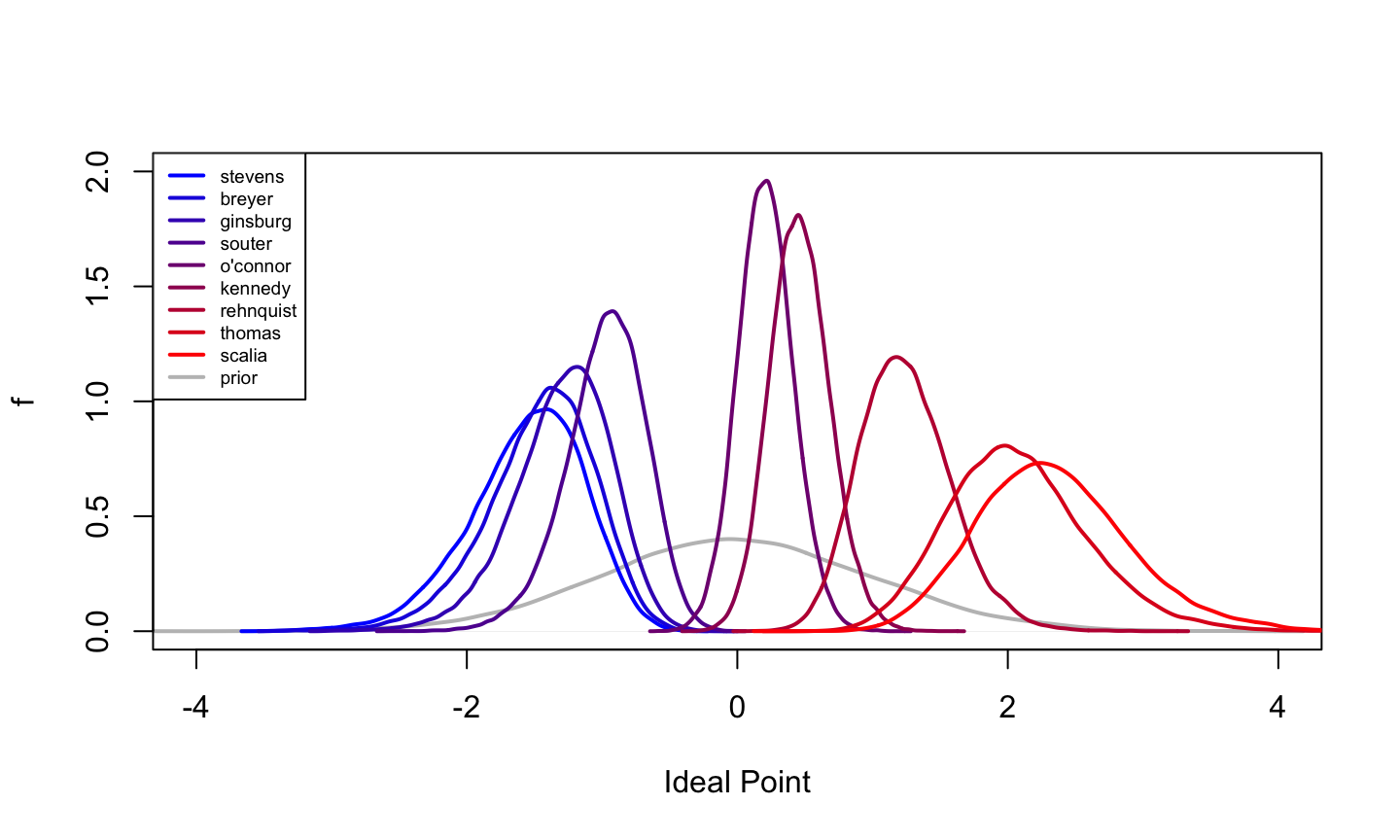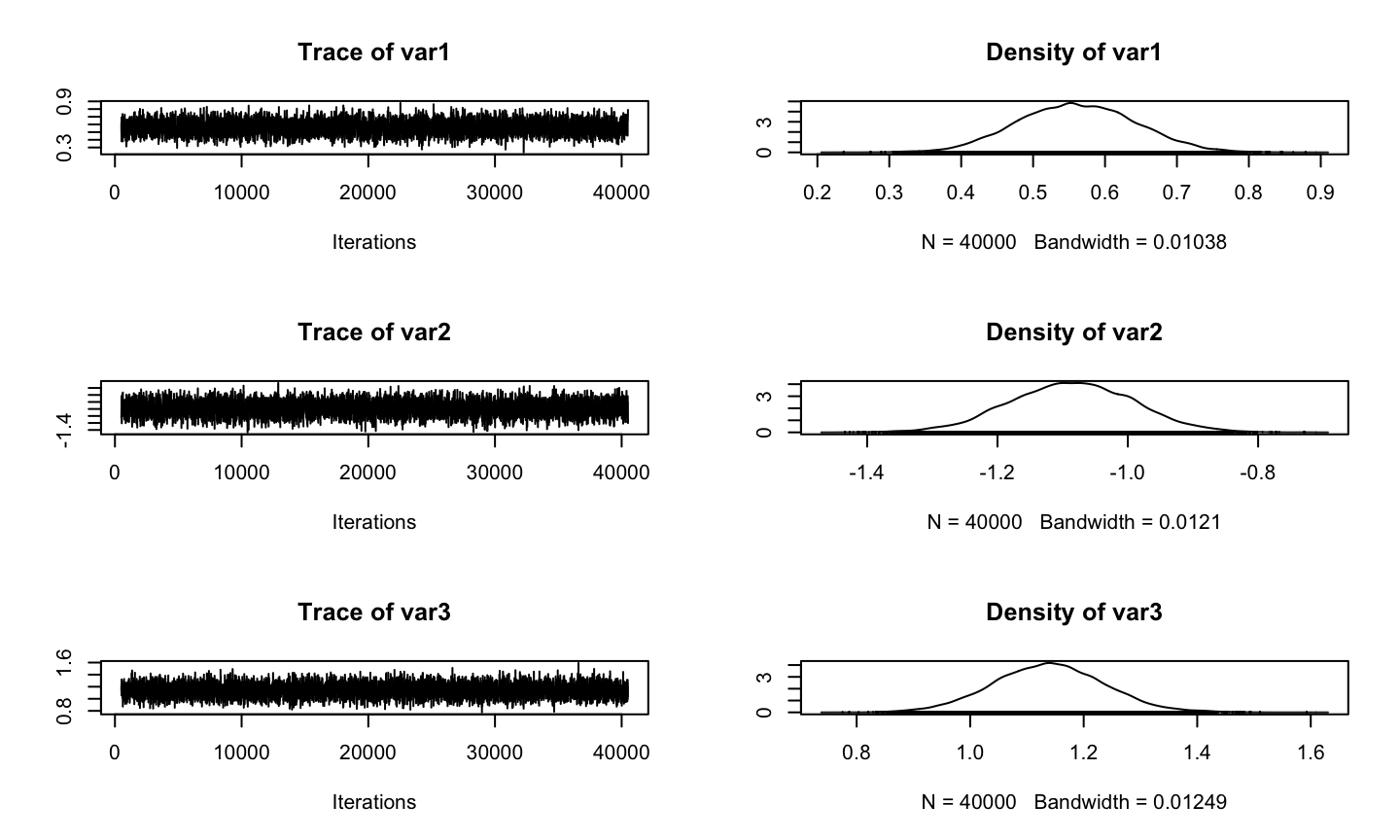## MCMCpack Introduction

• An R package that contains functions to perform Bayesian inference using posterior simulation.
• Aimed primarily at social scientists.
• The implementation of MCMC algorithms are model-specific.

## Functions in MCMCpack

Statistical Models:

• Linear regression models (linear regression with Gaussian errors, a singular value decomposition regression, and regression for a censored dependent variable)
• Discrete choice models (logistic/multinomial logistic/ordinal probit/probit regression)
• Measurement models (one-dimensional IRT,k-dimensional IRT, k-dimensional ordinal factor, k-dimensional linear factor, k-dimensional mixed factor, and k-dimensional robust IRT)
• A model for count data (Poisson regression model)
• Models for ecological inference (hierarchical ecological inference model and dynamic ecological inference model)
• Time-series models for change-point problems (binary/ probit/ ordinal probit/ Poisson change-point model).

Also contains some useful utility functions, including some additional density functions and pseudo-random number generators for statistical distributions, a general purpose Metropolis sampling algorithm, and tools for visualization.

## Installation of MCMCpack

install.packages(MCMCpack)
library(MCMCpack)
## Warning: package 'MCMCpack' was built under R version 3.4.3
## Warning: package 'MASS' was built under R version 3.4.3

## Ex1: Linear Regression Model

The model takes the following form:

$y_i = x_i^{'}\beta + \varepsilon_{i}, \quad \varepsilon_{i} \sim \mathcal{N}(0, 1/\tau)$

We assume standard, semi-conjugate priors:

$$\beta \sim \mathcal{N}(b_0,B_0^{-1})$$ and $$\tau \sim \mathcal{G}amma(c_0/2, d_0/2)$$

where $$\beta$$ and $$\tau$$ are assumed a priori independent.

MCMCregress(formula, data, burnin = 1000, mcmc = 10000, b0 = 0, B0 = 0,

marginal.likelihood = c("none", "Laplace", "Chib95"), ...)

burnin: The number of burn-in iterations for the sampler.

mcmc: The number of MCMC iterations after burnin.

b0: The prior mean of $$\beta$$.

B0: The prior precision of $$\beta$$.Default value of 0 is equivalent to an improper uniform prior for beta.

marginal.likelihood: "none" in which case the marginal likelihood will not be calculated, "Laplace" in which case the Laplace approximation is used, and "Chib95" in which case the method of Chib (1995) is used.

## Birthwt example

data(birthwt)
post.lm1 <- MCMCregress(bwt~age+lwt+as.factor(race) + smoke + ht,
data=birthwt,mcmc=50000,
b0=c(2700, 0, 0, -500, -500, -500, -500),
B0=c(1e-6, .01, .01, 1.6e-5, 1.6e-5, 1.6e-5,
1.6e-5),  marginal.likelihood="Chib95")
summary(post.lm1)
##
## Iterations = 1001:51000
## Thinning interval = 1
## Number of chains = 1
## Sample size per chain = 50000
##
## 1. Empirical mean and standard deviation for each variable,
##    plus standard error of the mean:
##
##                        Mean        SD  Naive SE Time-series SE
## (Intercept)        2766.216   266.744 1.193e+00      1.193e+00
## age                  -1.613     6.867 3.071e-02      3.071e-02
## lwt                   4.789     1.649 7.375e-03      7.375e-03
## as.factor(race)2   -497.656   129.948 5.811e-01      5.811e-01
## as.factor(race)3   -405.334   104.238 4.662e-01      4.662e-01
## smoke              -412.213    97.384 4.355e-01      4.355e-01
## ht                 -510.062   159.032 7.112e-01      7.112e-01
## sigma2           453136.179 47854.439 2.140e+02      2.182e+02
##
## 2. Quantiles for each variable:
##
##                        2.5%        25%        50%        75%      97.5%
## (Intercept)        2244.254   2586.351   2766.107   2944.028   3294.325
## age                 -15.057     -6.282     -1.654      3.061     11.908
## lwt                   1.545      3.686      4.785      5.897      8.034
## as.factor(race)2   -751.984   -584.729   -497.411   -410.831   -241.960
## as.factor(race)3   -610.201   -475.504   -405.602   -335.402   -201.463
## smoke              -604.018   -477.380   -412.223   -346.538   -220.036
## ht                 -822.476   -616.279   -510.280   -403.291   -197.281
## sigma2           368846.617 419405.802 450062.914 483105.313 555700.305

## Model Comparison

Suppose that the observed data y could have been generated under one of two models $$M_1$$ and $$M_2$$.

A natural thing to ask from the Bayesian perspective is, “what is the posterior probability that $$M_1$$ is true (assuming either $$M_1$$ or $$M_2$$ is true)?”

Using Bayes theorem we can write:

$Pr(M_k|y)=\frac{p(y|M_k)Pr(M_k)}{p(y|M_1)Pr(M_1)+p(y|M_1)Pr(M_1)}, \quad k=1,2$

$$p(y|M_1)$$ and $$p(y|M_2)$$ here are marginal liklihoods.

## Model Comparison

It is instructive to look at the posterior odds in favor of one model (say $$M_1$$): $\frac{Pr(M_1|y)}{Pr(M_2|y)}=\frac{p(y|M_1)}{p(y|M_2)} \times \frac{Pr(M_1)}{Pr(M_2)}$
Define Bayes factor for $$M_1$$ relative to $$M_2$$ as

$B_{12}=\frac{p(y|M_1)}{p(y|M_2)}$

Since the posterior odds equal the Bayes factor when the models are equally likely a priori, the Bayes factor is a measure of how much support is available in the data for one model relative to another.

## Model Comparison Example

PostProbMod: calculate the posterior probability that each model under study is correct given that one of the models under study is correct.

post.lm1 <- MCMCregress(bwt~age+lwt+as.factor(race) + smoke + ht,
data=birthwt,mcmc=50000,
b0=c(2700, 0, 0, -500, -500, -500, -500),
B0=c(1e-6, .01, .01, 1.6e-5, 1.6e-5, 1.6e-5,
1.6e-5),  marginal.likelihood="Chib95")
post.lm2 <- MCMCregress(bwt~age+lwt+as.factor(race) + smoke,
data=birthwt,mcmc=50000,
b0=c(2700, 0, 0, -500, -500, -500),
B0=c(1e-6, .01, .01, 1.6e-5, 1.6e-5, 1.6e-5),                                               marginal.likelihood="Chib95")
BF <- BayesFactor(post.lm1,post.lm2)
mod.probs <- PostProbMod(BF)

## Model Comparison Example

print(mod.probs)
##   post.lm1   post.lm2
## 0.93357327 0.06642673

## Ex2: Item Response Theory (IRT) Model

• $$y_{ij}$$ represents the observed choice by subject $$i$$ on item $$j$$.

• each subject has an ability (ideal point) dentoed by $$\theta_{i(K \times 1)}$$.

• each item has a difficulty parameter $$\alpha_j$$ and discrimination parameter $$\beta_{j(K \times 1)}$$.

Consider

$y_{ij} \sim Bernoulli(\pi_{ij}),\quad i=1,...,I \quad j=1,...,J$ $\pi_{ij}=\Phi (-\alpha_{j}+\beta_j^T\theta_i)$

where $$\Phi$$ is the the CDF of the standard normal distribution.

Prior distribution for the model parameters are: $(\alpha_j,\beta_j)^T \sim N(a_0,A_0^{-1}) \quad j=1,...,J$ and $\theta_i \sim N(0,1) \quad i=1,...,I$

MCMCirt1d(datamatrix, theta.constraints = list(), burnin = 1000, mcmc = 20000)
• datamatrix: The matrix of data. Must be 0, 1, or missing values. The rows of datamatrix correspond to subjects and the columns correspond to items.

• theta.constraints: A list specifying possible simple equality or inequality constraints on the ability parameters. A typical entry in the list has one of three forms:

(1). varname=c which will constrain the ability parameter for the subject named varname to be equal to c,

(2). varname="+" which will constrain the ability parameter for the subject named varname to be positive,

(3). varname="-" which will constrain the ability parameter for the subject named varname to be negative.

## SupremeCourt Example

• Data for 9 justices Rehnquist, Stevens, O'Connor, Scalia, Kennedy, Souter, Thomas, Ginsburg, and Breyer for the U.S. Supreme Court from 43 non-unanimous cases.
• The votes are coded liberal (1), conservative (0) or missing values.
data(SupremeCourt)
post.irt <- MCMCirt1d(t(SupremeCourt),
theta.constraints=list(Stevens="-",
Scalia="+"), burnin=5000, mcmc=100000)
summary(post.irt)
##
## Iterations = 5001:105000
## Thinning interval = 1
## Number of chains = 1
## Sample size per chain = 1e+05
##
## 1. Empirical mean and standard deviation for each variable,
##    plus standard error of the mean:
##
##                    Mean     SD  Naive SE Time-series SE
## theta.Rehnquist  1.2435 0.3380 0.0010689       0.008874
## theta.Stevens   -1.5566 0.4275 0.0013519       0.013209
## theta.O'Connor   0.2106 0.2040 0.0006450       0.004721
## theta.Scalia     2.3383 0.5590 0.0017678       0.020324
## theta.Kennedy    0.4647 0.2218 0.0007014       0.005229
## theta.Souter    -0.9754 0.2962 0.0009368       0.007523
## theta.Thomas     2.0760 0.5154 0.0016300       0.017308
## theta.Ginsburg  -1.2929 0.3603 0.0011395       0.010085
## theta.Breyer    -1.4533 0.4000 0.0012648       0.011456
##
## 2. Quantiles for each variable:
##
##                     2.5%      25%     50%     75%   97.5%
## theta.Rehnquist  0.64611  1.00643  1.2227  1.4582  1.9691
## theta.Stevens   -2.49232 -1.81881 -1.5167 -1.2525 -0.8294
## theta.O'Connor  -0.18238  0.07256  0.2072  0.3441  0.6223
## theta.Scalia     1.34693  1.94596  2.3028  2.6873  3.5606
## theta.Kennedy    0.04852  0.31259  0.4575  0.6090  0.9206
## theta.Souter    -1.62223 -1.15759 -0.9554 -0.7687 -0.4555
## theta.Thomas     1.17013  1.71491  2.0401  2.3928  3.1980
## theta.Ginsburg  -2.08412 -1.51247 -1.2621 -1.0394 -0.6743
## theta.Breyer    -2.33480 -1.69472 -1.4166 -1.1738 -0.7753## EX3:Metropolis Sampling for a User-Defined Model

MCMCpack also has some facilities for fitting user-specified models.

The MCMCmetrop1R() function uses a random walk Metropolis algorithm to sample from a user-defined log-posterior density.

MCMCmetrop1R(fun, theta.init, burnin = 500, mcmc = 20000,
tune = 1, logfun = TRUE, force.samp = FALSE,
V = NULL, optim.method = "BFGS", ...)

fun: The unnormalized (log)density of the distribution from which to take a sample.

theta.init: Starting values for the sampling. Must be of the appropriate dimension. It must also be the case that fun(theta.init, …) is greater than -Inf if fun() is a logdensity or greater than 0 if fun() is a density.

tune: The tuning parameter for the Metropolis sampling. Can be either a positive scalar or a k-vector, where k is the length of θ.

## Example for a User-Defined Model

Suppose one is interested in fitting a logistic regression with an improper uniform prior.

One could do the following:

 logitfun <- function(beta, y, X){
eta <- X %*% beta
p <- 1.0/(1.0+exp(-eta))
sum( y * log(p) + (1-y)*log(1-p) )
}

x1 <- rnorm(1000)
x2 <- rnorm(1000)
Xdata <- cbind(1,x1,x2)
p <- exp(.5 - x1 + x2)/(1+exp(.5 - x1 + x2))
yvector <- rbinom(1000, 1, p)
post.samp <- MCMCmetrop1R(logitfun, theta.init=c(0,0,0),
X=Xdata, y=yvector,
mcmc=40000, burnin=500,
tune=c(1.5, 1.5, 1.5),
logfun=TRUE)
##
##
## @@@@@@@@@@@@@@@@@@@@@@@@@@@@@@@@@@@@@@@@@@@@@@@@@@@@@@@@@
## The Metropolis acceptance rate was 0.28891
## @@@@@@@@@@@@@@@@@@@@@@@@@@@@@@@@@@@@@@@@@@@@@@@@@@@@@@@@@
plot(post.samp)summary(post.samp)
##
## Iterations = 501:40500
## Thinning interval = 1
## Number of chains = 1
## Sample size per chain = 40000
##
## 1. Empirical mean and standard deviation for each variable,
##    plus standard error of the mean:
##
##        Mean      SD  Naive SE Time-series SE
## [1,]  0.561 0.08153 0.0004076       0.001298
## [2,] -1.086 0.09502 0.0004751       0.001552
## [3,]  1.139 0.09813 0.0004906       0.001589
##
## 2. Quantiles for each variable:
##
##         2.5%     25%     50%     75%   97.5%
## var1  0.4055  0.5041  0.5597  0.6165  0.7208
## var2 -1.2748 -1.1511 -1.0854 -1.0206 -0.9002
## var3  0.9469  1.0724  1.1379  1.2038  1.3343